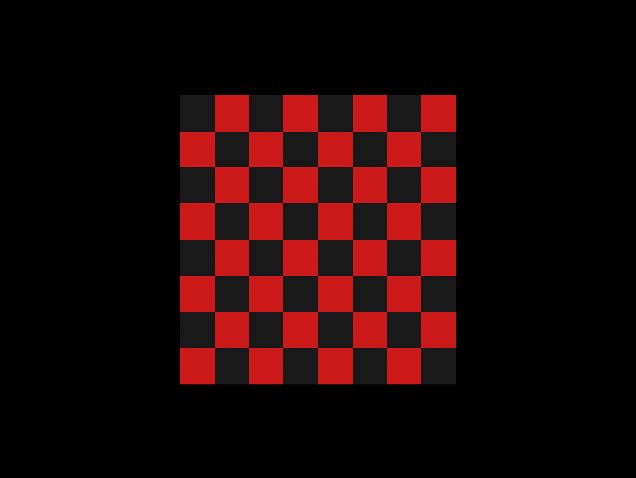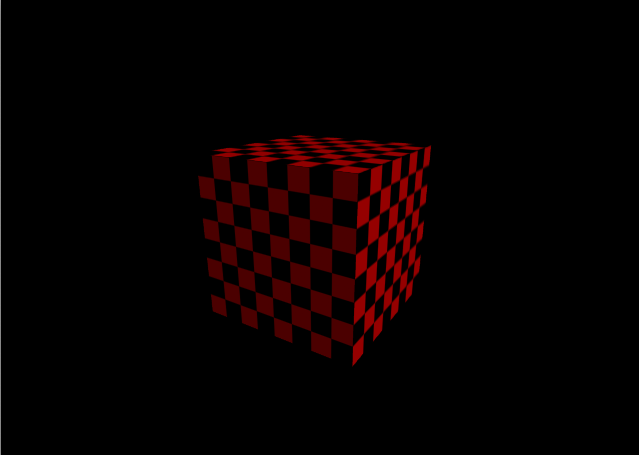«

# rendering textures in clojure

## 2009

At its simplest, a texture is an image stretched across a shape. We can define a texture like so:

``````(def checkers (create-color-texture 128 128))

(draw-to-subsampled-texture
checkers
(fn [[x y] _]
(if (xor (even? (int (/ x 16))) (even? (int (/ y 16))))
[0.8 0.1 0.1 1]
[0.1 0.1 0.1 1]))))
``````

First we create a texture that is 128x128 pixels. Then we populate the texture using a function that returns a color for each individual pixel. We render the texture by associating two-dimensional coordinates in texture-space with each vertex. These coordinates are independent of any specific texture. To associate them with the texture we just defined, we must call bind-texture.

``````(defn textured-quad []
(push-matrix
(translate -0.5 -0.5 0.5)
(normal 0 0 -1)
(texture 1 1) (vertex 1 1 0)
(texture 0 1) (vertex 0 1 0)
(texture 0 0) (vertex 0 0 0)
(texture 1 0) (vertex 1 0 0))))

(bind-texture checkers)
``````We can apply the same texture to multiple shapes. Wherever a textured quad is drawn, the texture that is currently bound will be drawn across it.

``````(defn textured-cube []

;; draw the four sides
(dotimes [_ 4]
(rotate 90 0 1 0)

;; draw the top
(rotate 90 1 0 0)

;; draw the bottom
(rotate 180 1 0 0)

(bind-texture checkers)
(textured-cube)
``````This rendering of a cube can itself be made into a texture, which can be applied to another shape.

``````(def scene (create-texture 128 128))

(render-to-texture scene
(with-projection (frustum-view 50 1 0.1 10)
(textured-cube)))
``````

Putting everything together, we get this:

These examples use the Penumbra library. Full code can be found in the `/examples` subdirectory.